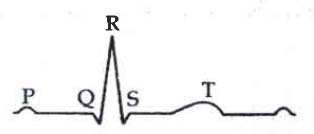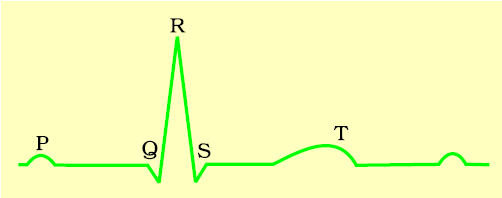Q

# Solve this problem The diagram given here is the standard ECG of a normal person. The P - wave represents the:

The diagram given here is the standard ECG of a normal person. The P - wave represents the:• Option 1)

End of systole

• Option 2)

Contraction of both the atria

• Option 3)

Initiation of the ventricular contraction

• Option 4)

Beginning of the systole

206 Views

Steps to obtain a standard ECG -

Each peak in the ECG is identified with a letter from P to T that corresponds to a specific electrical activity of the heart.

- whereinP - wave represents contraction of both atria, which is associated with both left and right atrial depolarization

Option 1)

End of systole

Option is Incorrect

Option 2)

Contraction of both the atria

Option is Correct

Option 3)

Initiation of the ventricular contraction

Option is Incorrect

Option 4)

Beginning of the systole

Option is Incorrect

Exams
Articles
Questions